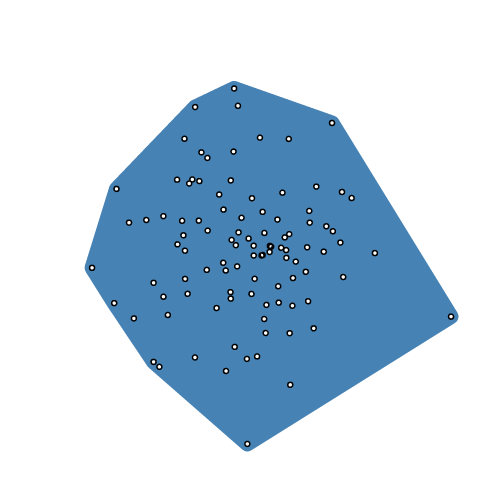/D3.js 5

# d3-polygon

This module provides a few basic geometric operations for two-dimensional polygons. Each polygon is represented as an array of two-element arrays [​[x1, y1], [x2, y2], …], and may either be closed (wherein the first and last point are the same) or open (wherein they are not). Typically polygons are in counterclockwise order, assuming a coordinate system where the origin ⟨0,0⟩ is in the top-left corner.

## Installing

If you use NPM, `npm install d3-polygon`. Otherwise, download the latest release. You can also load directly from d3js.org, either as a standalone library or as part of D3 4.0. AMD, CommonJS, and vanilla environments are supported. In vanilla, a `d3` global is exported:

```<script src="https://d3js.org/d3-polygon.v1.min.js"></script>
<script>

var hull = d3.polygonHull(points);

</script>```

## API Reference

###### d3.polygonArea(polygon) <>

Returns the signed area of the specified polygon. If the vertices of the polygon are in counterclockwise order (assuming a coordinate system where the origin ⟨0,0⟩ is in the top-left corner), the returned area is positive; otherwise it is negative, or zero.

###### d3.polygonCentroid(polygon) <>

Returns the centroid of the specified polygon.

###### d3.polygonHull(points) <>Returns the convex hull of the specified points using Andrew’s monotone chain algorithm. The returned hull is represented as an array containing a subset of the input points arranged in counterclockwise order. Returns null if points has fewer than three elements.

###### d3.polygonContains(polygon, point) <>

Returns true if and only if the specified point is inside the specified polygon.

###### d3.polygonLength(polygon) <>

Returns the length of the perimeter of the specified polygon.